This page has been robot translated, sorry for typos if any. Original content here.

 home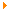EconomyBooksModel of economics - Vіtlіnsky V.V.

# Model of economics - Vіtlіnsky V.V.

## 6.4.Model and method for calculating the EU rating

Adequate mathematical model for the analysis of the set of indicators є system S , which can be seen as an archetype. Be-yak methodology for ranking ranking is reduced to the last factorization set of n vihdnyh indicators, the result of such an element is linearly ordered (multiple) multiples. I’ll unify the description of the process of calculating the rating on the preliminary analysis of complex assessments (irrespective of the specific methodology), but I can have a little more room at the discrete view of the reconsideration M. The area of ​​such reinterpretation is є n- mirniy masiv A , de p is the number of victorious complex characteristics.

The leather index is one of the most comprehensive characteristics, and the value of the index is the valid value of the characteristics (numbered by the standard scale).

Peretvoryuvach M funktsionuє such an order. Yakshcho kіlkіst vimіryuvanih characteristics of the roadway rozmіrnostі masivu, then M is just a healthy way to vibrate the element masivu. As a matter of fact, I’ve enumerated the characteristics of the mensch, nizzh rozmіrnіst masiva, then overturning M function so such a rank.

Nekhai set nabir value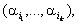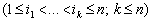Characteristics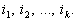Reacting overturning of M for entrance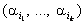є so three numbers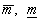that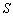, scho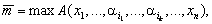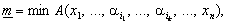de maximum i minimum take on all permissible values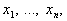and S is the average value (to mathematically match) for all admissible values. The numbers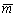that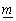to name one optimistic and one rating. In the fake tri_yka (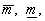S ) Much but the number has been redone in the same way as before the methodology.

An important role is played by the transition of the S system to the re-engineering of the M -method of forecasting (both statistical and expert) and the classification of economic objects (cluster analysis).